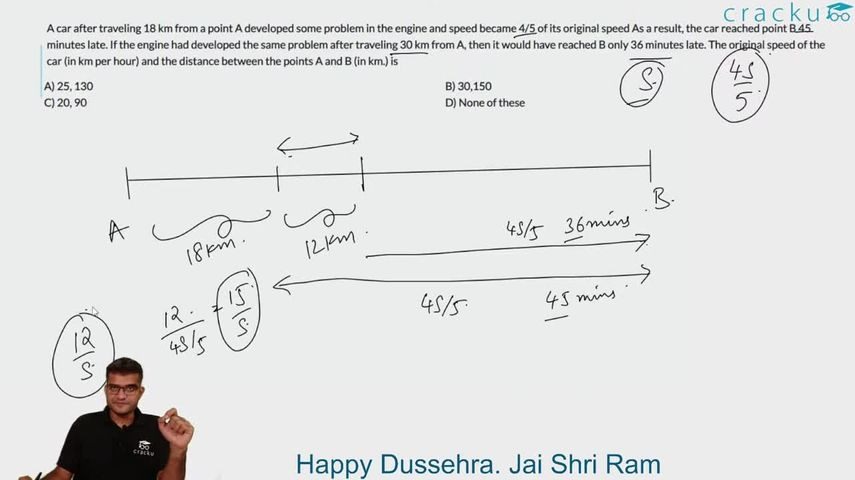Question 36

# A car after traveling 18 km from a point A developed some problem in the engine and speed became 4/5 of its original speed As a result, the car reached point B 45 minutes late. If the engine had developed the same problem after traveling 30 km from A, then it would have reached B only 36 minutes late. The original speed of the car (in km per hour) and the distance between the points A and B (in km.) is

Solution

Time difference, when second time car's engine failed at a distance of 30 km., is of 9 min.
Hence putting this in equation:
$$\frac{12}{\frac{4v}{5}} - \frac{12}{v} = \frac{9}{60}$$ hr. (Because difference of time is considered with extra travelling of 12 km. in second case)
We will get $$v (velocity) = 20$$ km/hr.
Now for distance $$\frac{d-18}{16} + \frac{18}{20} - \frac{d}{20} = \frac{45}{60}$$ hr. (As car is 45 min. late after engine's faliure in first case)
So $$d$$ = 78 km.
Hence none of these will be our answer.

### View Video Solution